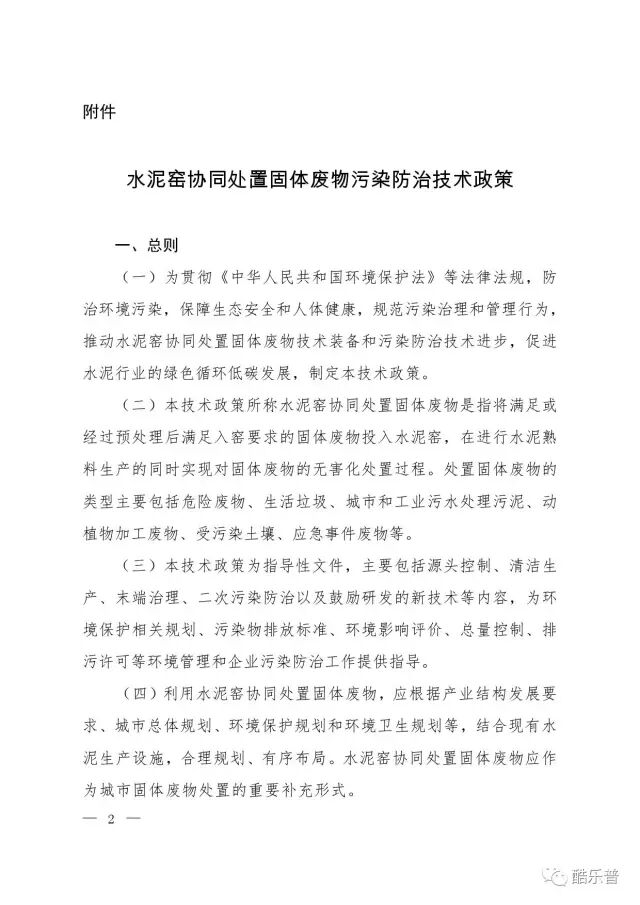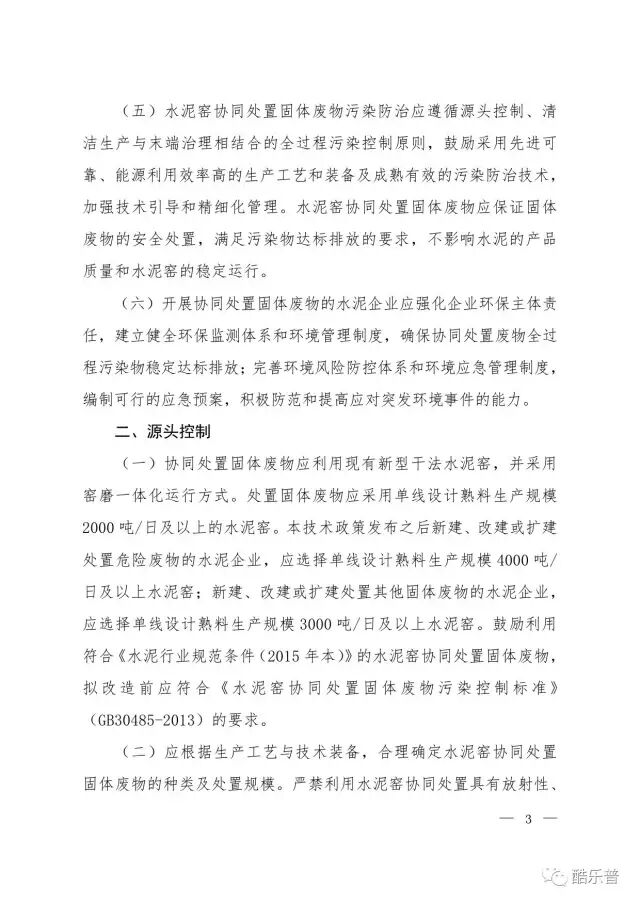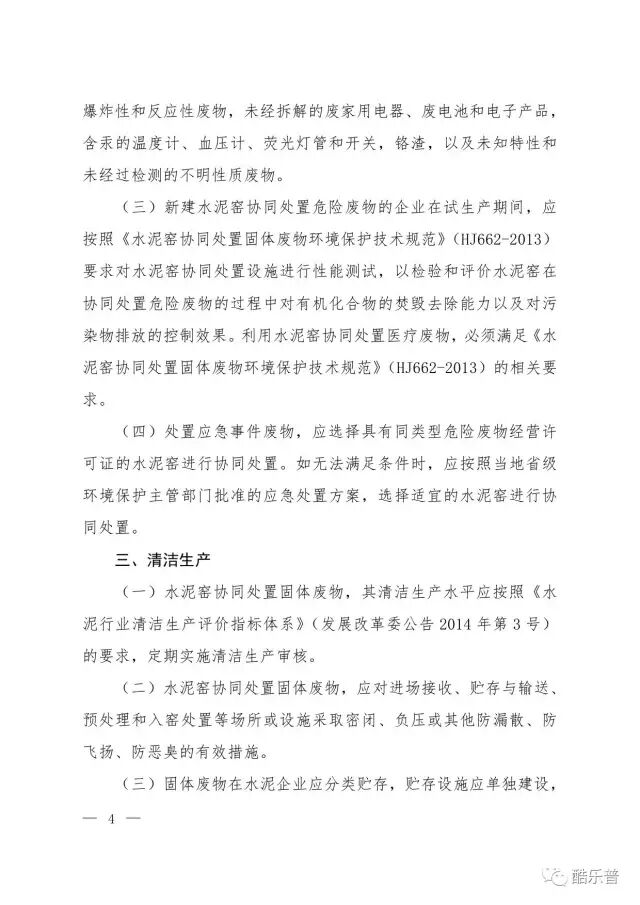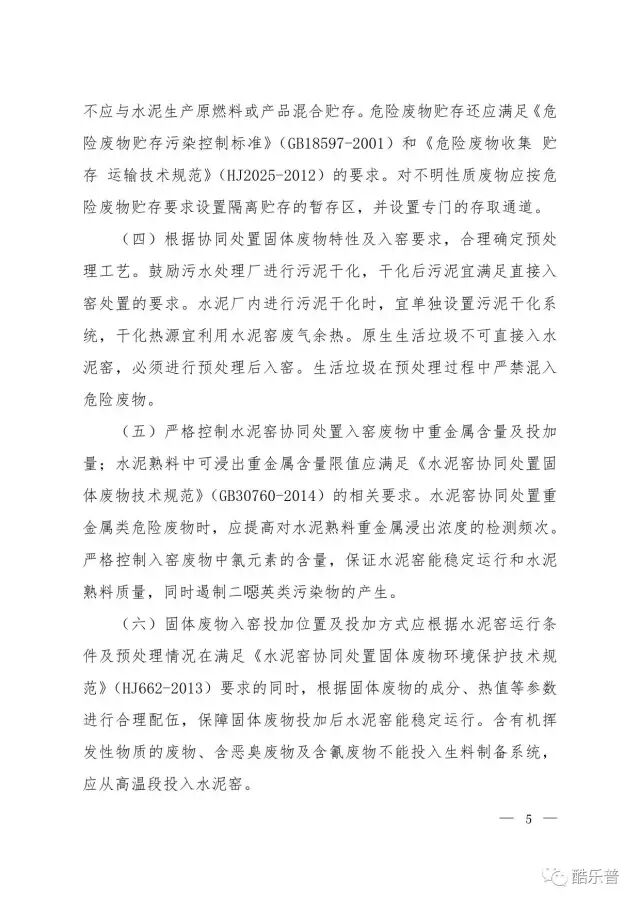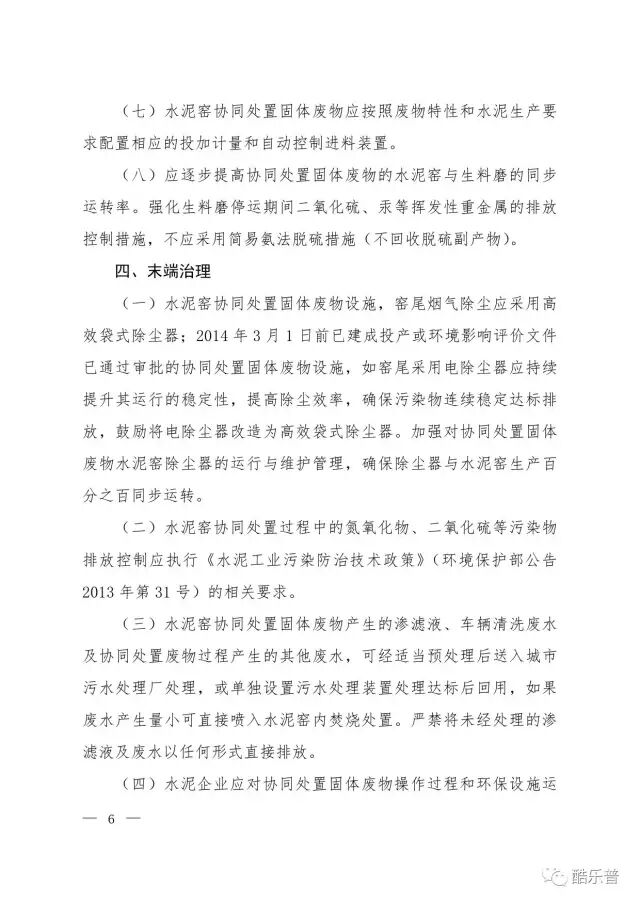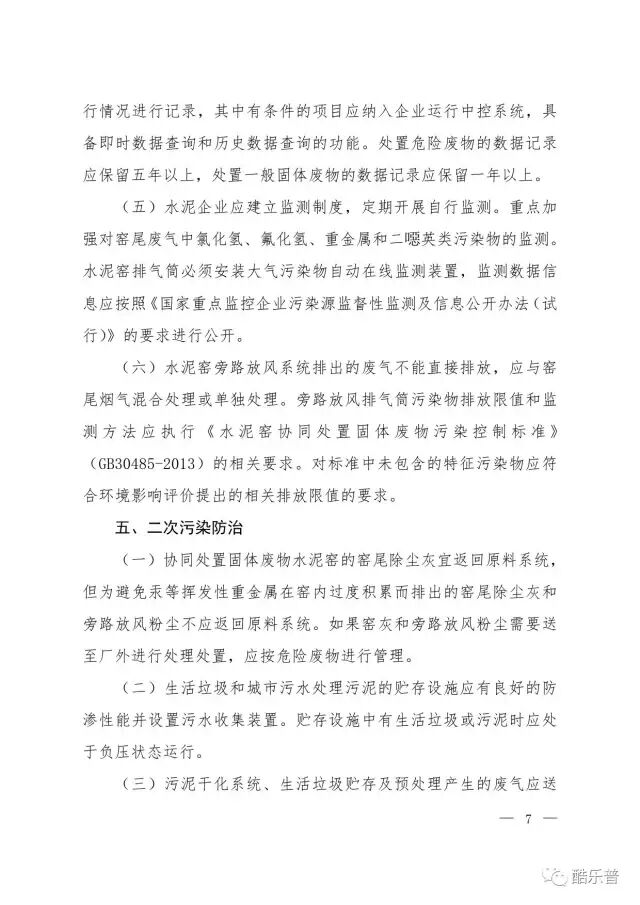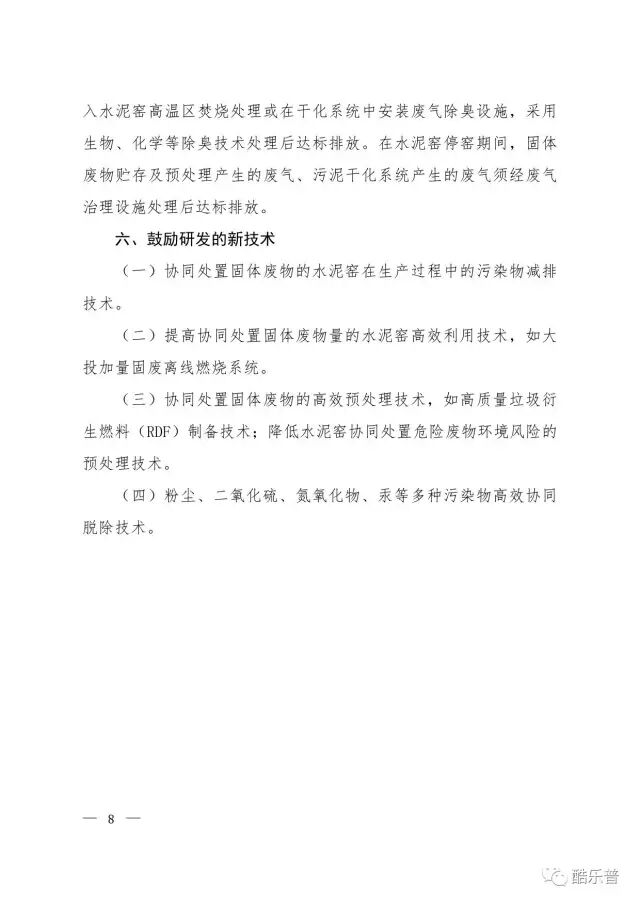#### 今天是 function showLocale(objD) { var str,colorhead,colorfoot; var yy = objD.getYear(); if(yy<1900) yy = yy+1900; var MM = objD.getMonth()+1; if(MM<10) MM = '0' + MM; var dd = objD.getDate(); if(dd<10) dd = '0' + dd; var hh = objD.getHours(); if(hh<10) hh = '0' + hh; var mm = objD.getMinutes(); if(mm<10) mm = '0' + mm; var ss = objD.getSeconds(); if(ss<10) ss = '0' + ss; var ww = objD.getDay(); if ( ww==0 ) colorhead="<font color=\"#333333\">"; if ( ww > 0 && ww < 6 ) colorhead="<font color=\"#333333\">"; if ( ww==6 ) colorhead="<font color=\"#333333\">"; if (ww==0) ww="星期日"; if (ww==1) ww="星期一"; if (ww==2) ww="星期二"; if (ww==3) ww="星期三"; if (ww==4) ww="星期四"; if (ww==5) ww="星期五"; if (ww==6) ww="星期六"; colorfoot="</font>" //str = colorhead + yy + "年" + MM + "月" + dd + "日"+ " " + ww + " " + hh + ":" + mm + ":" + ss + colorfoot; str = colorhead + yy + "年" + MM + "月" + dd + "日"+ " " + ww + colorfoot; return(str); } function tick() { var today; today = new Date(); document.getElementById("localtime").innerHTML = showLocale(today); window.setTimeout("tick()", 1000); } tick(); 返回首页 丨联系方式### 关于发布《水泥窑协同处置固体废物污染防治技术政策》的公告

（公告 201672号）

2016126